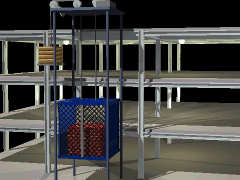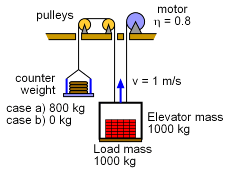Ch 3. Particle Energy Methods Multimedia Engineering Dynamics Work &Energy Conservative Forces Power & Efficiency
 Chapter - Particle - 1. General Motion 2. Force & Accel. 3. Energy 4. Momentum - Rigid Body - 5. General Motion 6. Force & Accel. 7. Energy 8. Momentum 9. 3-D Motion 10. Vibrations Appendix Basic Math Units Basic Equations Sections Search eBooks Dynamics Fluids Math Mechanics Statics Thermodynamics Author(s): Kurt Gramoll ©Kurt GramollDYNAMICS - CASE STUDY IntroductionProblem Graphic A construction worker on the new city hall wonders why the elevator has a counterbalance. Does a counterbalance affect the power needed to operate the elevator? What is known: elevator mass me = 1000 kg elevator load mc = 1000 kg motor efficiency e = 0.8 elevator speed ve = 1 m/s Assume that the elevator moves with constant velocity and all transient accelerations can be ignored.Problem Diagram Question What power (kilowatts) must the elevator motor produce to lift the elevator with an 800 kg counterweight? no counterweight? Approach Power is simply work per time, or   P = ΔU/Δt For computations, use   P = F·v

Practice Homework and Test problems now available in the 'Eng Dynamics' mobile app
Includes over 400 problems with complete detailed solutions.
Available now at the Google Play Store and Apple App Store.